Question

A particle starts from the origin (0,0) and moves to position (9,16) in 1.5s. If the particle starts from rest, what is the acceleration in component form of the particle?

The particle starts from rest, u =0m/s

A particle starts from the origin (0,0) and moves to position (9,16)

so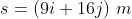Use formula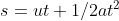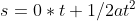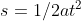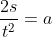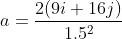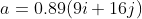ANSWER: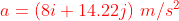============================

#### Earn Coins

Coins can be redeemed for fabulous gifts.

Similar Homework Help Questions
• ### A particle starts from rest at the origin with an acceleration vector that has magnitude 6...

A particle starts from rest at the origin with an acceleration vector that has magnitude 6 m/s2 and direction 29 ∘ above the positive x axis. What is the vx component of its velocity vector 25 s later? What is the vy component of its velocity vector 25 s s later? What is the particle’s dx position at that time? What is the particle’s dy position at that time?

• ### A particle moves in a straight line with the acceleration shown. The particle starts from the...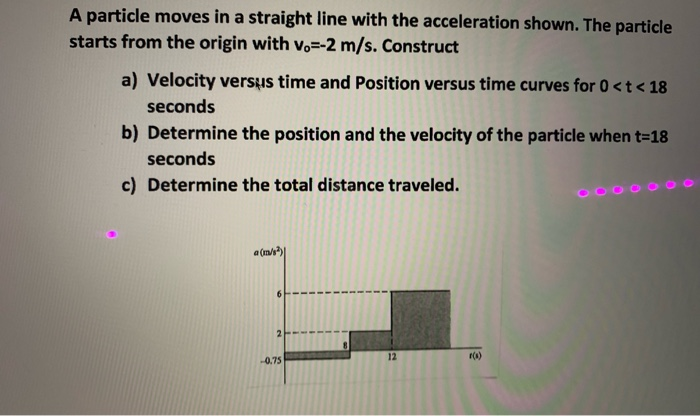A particle moves in a straight line with the acceleration shown. The particle starts from the origin with V.=-2 m/s. Construct a) Velocity versus time and Position versus time curves for 0 <t< 18 seconds b) Determine the position and the velocity of the particle when t=18 seconds c) Determine the total distance traveled. ooo a( )

• ### A particle with 500 gm mass starts from rest and moves by a force F(x) =...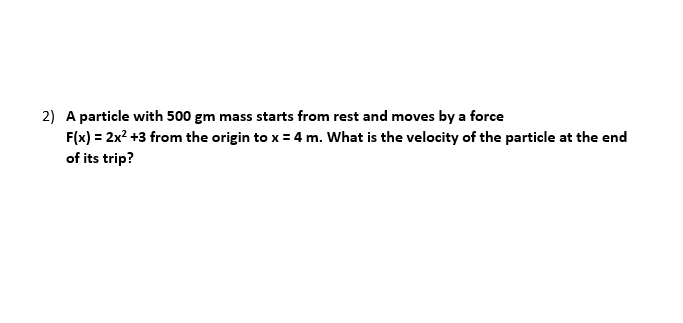A particle with 500 gm mass starts from rest and moves by a force F(x) = 2x2 +3 from the origin to x = 4 2) A particle with 500 gm mass starts from rest and moves by a force F(x) = 2x2 +3 from the origin to x = 4 m. What is the velocity of the particle at the end of its trip?

• ### A particle starts from the origin at t Owith a velocity of 6.2j and moves in...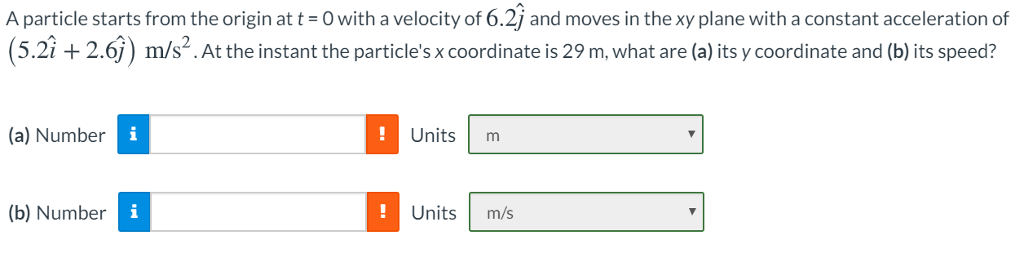A particle starts from the origin at t Owith a velocity of 6.2j and moves in the xy plane with a constant acceleration of (5.2i +2.6j) m/s2. At the instant the particle's x coordinate is 29 m, what are (a) its y coordinate and (b) its speed? (a) Number Unitsm (b) Number Units m/s

• ### Determine the work done by a particle that moves from the P position (0,0) to the...

Determine the work done by a particle that moves from the P position (0,0) to the Q position (1,0) in the vector field: F (x, y) = e^3y i + (-3e^3y x + y) j

• ### A particle starts from rest at x = -1.8 m and moves along the x-axis with...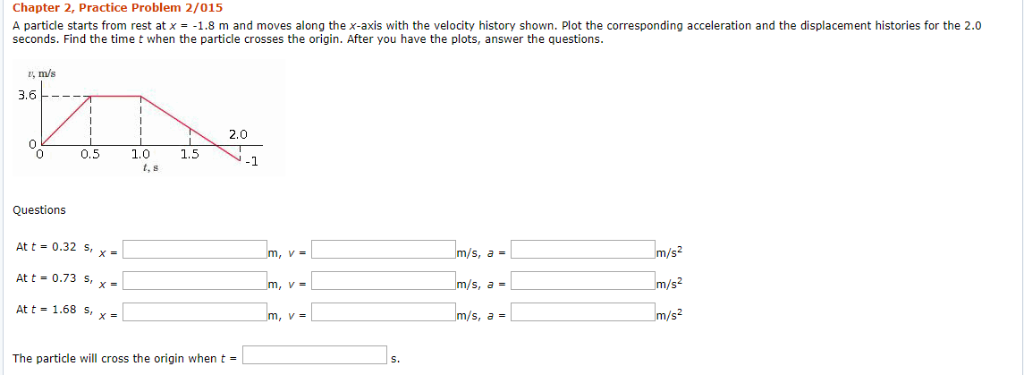A particle starts from rest at x = -1.8 m and moves along the x-axis with the velocity history shown. Plot the corresponding acceleration and the displacement histories for the 2.0 seconds. Find the time t when the particle crosses the origin. After you have the plots, answer the questions. Chapter 2, Practice Problem 2/015 A particle starts from rest at x = -1.8 m and moves along the x-axis with the velocity history shown. Plot the corresponding acceleration and...

• ### Suppose that a particle starts at the origin of the real line and moves along the...

Suppose that a particle starts at the origin of the real line and moves along the line in jumps of one unit. For each jump, the probability is p(0≤p≤1) that the particle will jump one unit to the left and the probability is 1−p that the particle will jump one unit to the right. Find the expected value of the position of the particle after n jumps.

• ### At t = 0 s, a particle starts from the origin of a coordinate system and...

At t = 0 s, a particle starts from the origin of a coordinate system and moves in the xy plane with a velocity ~v = (7.9ˆi − 3.2ˆj) m/s. Determine the x position of the particle at t = 2.0 s.

• ### a particle starts at time t=0 and moves on a number line so that its position at time t is given by x(t)= (t-2)^3(t-6) what is the farthest to the left of the origin that hte particles moves

a particle starts at time t=0 and moves on a number line so that its position at time t is given by x(t)= (t-2)^3(t-6) what is the farthest to the left of the origin that hte particles moves?? i was able to find the velocity and acceleration formula. but i have no idea how to do this

• ### A particle starts from the origin at t = 0 and moves along the positive x axis. A graph of the velocity of the particle as a function of the time is shown in the figure;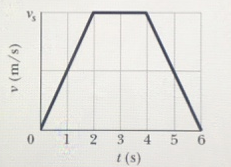A particle starts from the origin at t = 0 and moves along the positive x axis. A graph of the velocity of the particle as a function of the time is shown in the figure; the v-axis scale is set by vs = 6.0 m/s. (a) what is the coordinate of the particle at t = 5.0 s? (b) what is the velocity of the particle at t = 5.0 s? (c) what is the acceleration of the particle...